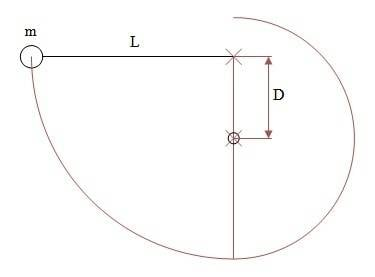# Mass attached to a rope. the rope encounters a nail.

Karol

## Homework Statement

M is attached to a rope L and starts from horizontal. the rope hits a nail at distance D.
What is m's velocity when it reaches the lowest point.
At what minimal distance D should the nail be places so that m will complete a circle round the nail.## Homework Equations

Kinetic energy: ##E_k=\frac{1}{2}mv^2##
Potential energy: ##E_p=mgh##
Centrifugal force: ##F=m\frac{v^2}{r}##

## The Attempt at a Solution

Conservation of energy relative to the lowest point:
$$mgL=\frac{1}{2}mv^2\;\rightarrow\; v=\sqrt{2gL}$$
The centrifugal force at the top equals only the weight:
$$m\frac{v_2^2}{L-D}=mg\;\rightarrow\;v_2^2=(L-D)g$$
The kinetic energy at the bottom, which equals the initial potential energy, equals the potential and kinetic energy at the top:
$$\frac{1}{2}mg(L-D)+2\cdot mg(L-D)=mgL\;\rightarrow\; D=\frac{3}{5}L$$

Homework Helper

## Homework Statement

M is attached to a rope L and starts from horizontal. the rope hits a nail at distance D.
What is m's velocity when it reaches the lowest point.
At what minimal distance D should the nail be places so that m will complete a circle round the nail.
View attachment 99213

## Homework Equations

Kinetic energy: ##E_k=\frac{1}{2}mv^2##
Potential energy: ##E_p=mgh##
Centrifugal force: ##F=m\frac{v^2}{r}##

## The Attempt at a Solution

Conservation of energy relative to the lowest point:
$$mgL=\frac{1}{2}mv^2\;\rightarrow\; v=\sqrt{2gL}$$
The centrifugal force at the top equals only the weight:
$$m\frac{v_2^2}{L-D}=mg\;\rightarrow\;v_2^2=(L-D)g$$
The kinetic energy at the bottom, which equals the initial potential energy, equals the potential and kinetic energy at the top:
$$\frac{1}{2}mg(L-D)+2\cdot mg(L-D)=mgL\;\rightarrow\; D=\frac{3}{5}L$$
It looks correct.

Karol
Thank you Ehild